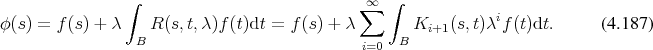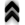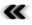### 4.9 Integral Equations

The integral equation under consideration in this context is the Fredholm integral equation of the second kind, which is commonly given in the form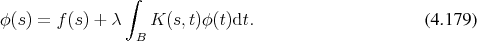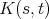is the kernel of the integral equation. As the solution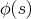appears on both sides of Equation 4.179, it can be inserted into itself, thus yielding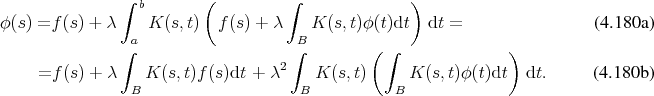Thus the function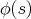takes the form of a series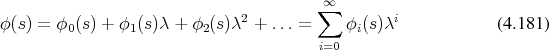where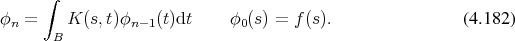While this recursion relation is straightforward, an explicit expression for the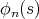depending only on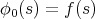is favourable. It can be obtained by rewriting the recursion with a focus on the kernels instead of the functions to read.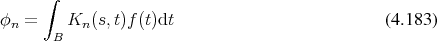Now the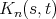are given as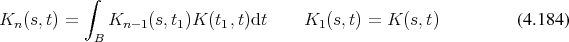which can be generalized to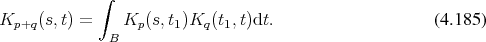This representation is known as iterated kernels. The resulting series is known as a Neumann series or the resolvent series of the Equation 4.179 and takes the form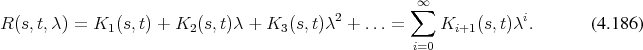Thus the expression of Equation 4.181 may also be expressed as